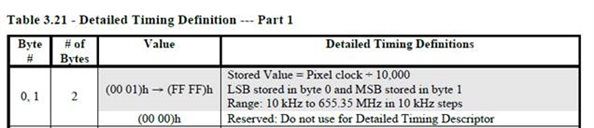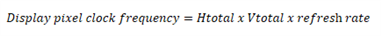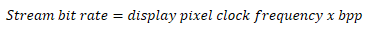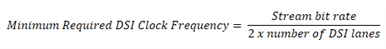If you have a related question, please click the "Ask a related question" button in the top right corner. The newly created question will be automatically linked to this question.

# [FAQ] SN65DSI86: SN65DSI86 Resolution Guide

Part Number: SN65DSI86

What display resolution will the SN65DSI86 support?

• The way to know whether or not the SN65DSI86 will support your display panel resolution is by determining the minimum required DSI clock frequency needed. The MIPI DSI input of the DSI86 can support frequencies up to 750MHz.

To find the minimum required DSI clock frequency, you will need to perform a simple calculation to find the stream bit rate. The stream bit rate shows the rate at which bits are transferred to the display. To perform this calculation, you will first need to locate the display pixel clock frequency. The display EDID should show the supported pixel clock frequency, it is normally located in the detailed timing definition portion of the EDID.For example, in this EDID, a pixel clock of 135MHz would be represented by 13500 decimal which is stored as BCh, 34h.

Now, what if you can't locate the pixel clock frequency from the EDID? In this case, if you at least know Htotal, Vtotal, and the desired refresh rate, then you can estimate what DSI CLK frequency will be required by using the equation below:Once you have performed this calculation, you can use the pixel clock estimation to perform the stream bit rate and minimum DSI CLK equations below. Please only use the display pixel clock frequency estimation if you cannot locate the actual pixel clock frequency in the display EDID.

The stream bit rate is a function of the eDP display pixel clock frequency and the amount of bits per pixel (bpp), shown below.Assuming 24bpp and a pixel clock frequency of 135MHz, the stream bit rate would be 3.24GHz. The stream bit rate is used to calculate the minimum required DSI clock frequency needed on the DSI86 to support the desired resolution. Note that the Max DSI Clock frequency supported by the DSI86 is 750MHz. The DSI86 can support up to 8 DSI data lanes.With a stream bit rate of 3.24GHz, and assuming all 8 lanes are being used, the minimum required DSI clock frequency would be 202.5MHz. This frequency is well within the 750MHz limit of the DSI86 supported DSI clock frequency, so this panel would be supported.